# RD Sharma Solutions for Class 8 Maths Chapter 26 Data Handling - IV (Probability)

The word probability is commonly used in our day-to-day conversation like it may probably rain today, he is probably right, he may probably join games etc. However, in the theory of probability, we assign a numerical value to the degree of uncertainty. ‘Probability’ has emerged as one of the basic tools of statistics today and has a wide range of applications in science and engineering. We shall see all these discussions in Chapter 26. For quick reference, students can refer to RD Sharma Solutions. The solutions of this exercise are available in the RD Sharma Class 8 Maths Chapter 26 Exercise 26.1, in the pdf format, which is provided in the links below.

Chapter 26 Data Handling – IV (Probability) contains one exercise and the RD Sharma Class 8 Solutions present in this page provide solutions to the questions present in this exercise. Now, let us have a look at the concepts discussed in this chapter.

• A theoretical approach to probability.
• Elementary event.
• Compound event.
• The occurrence of an event.
• Favourable elementary events
• The negation of an event.
• Theoretical probability.

## Download the Pdf of RD Sharma Solutions for Class 8 Maths Chapter 26 Data Handling – IV (Probability)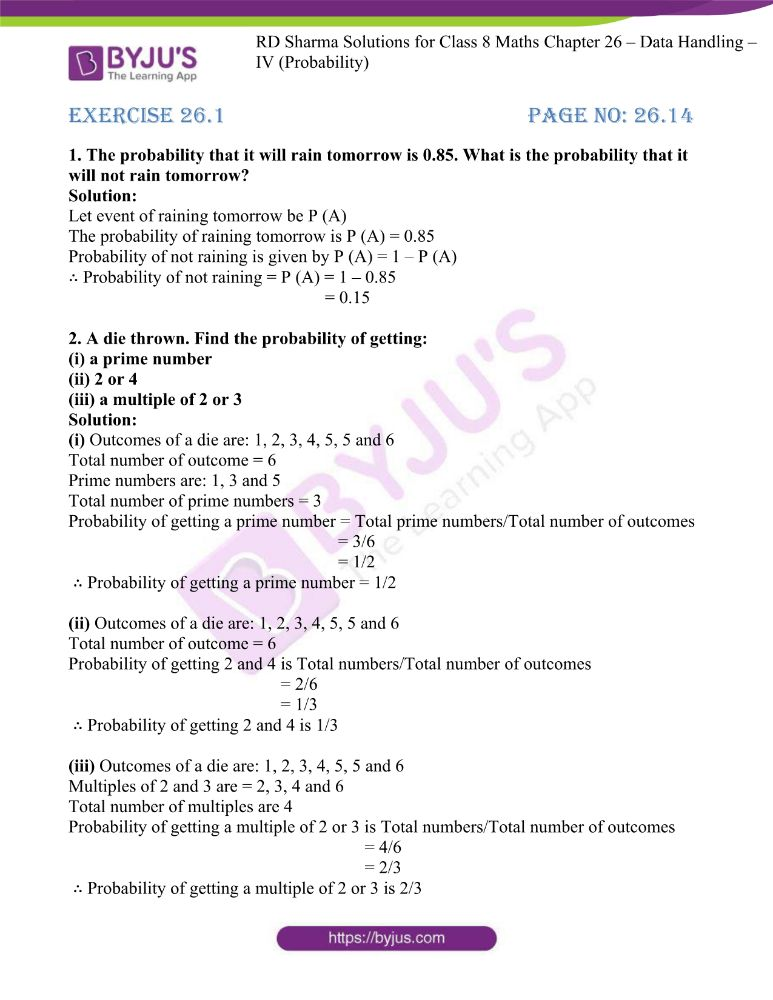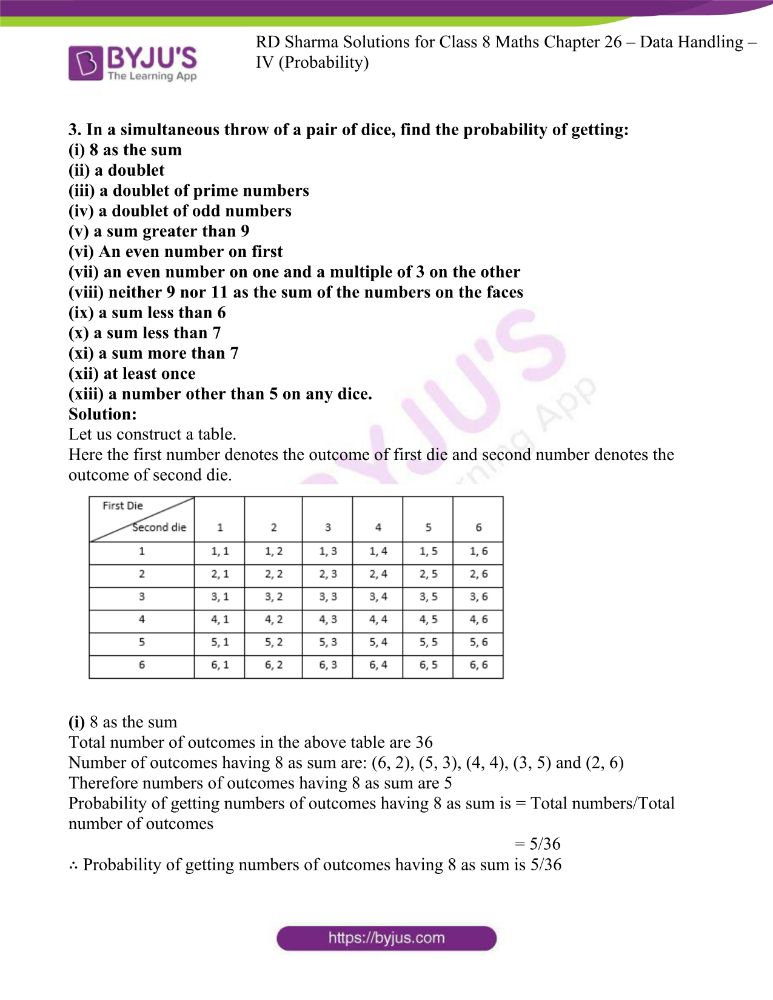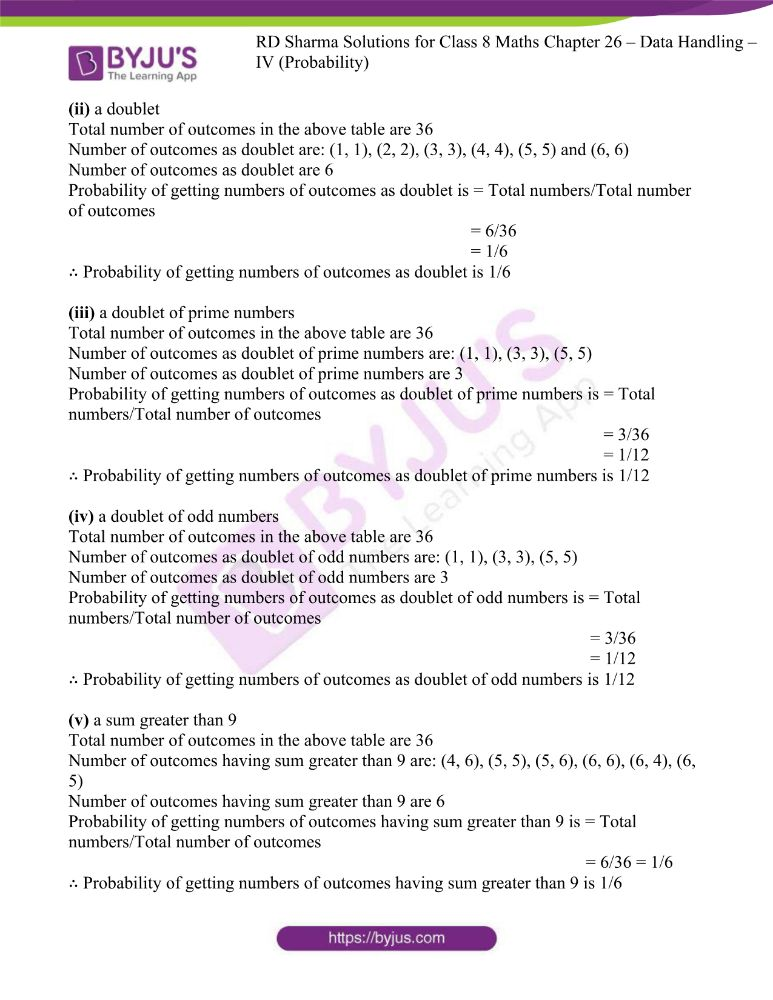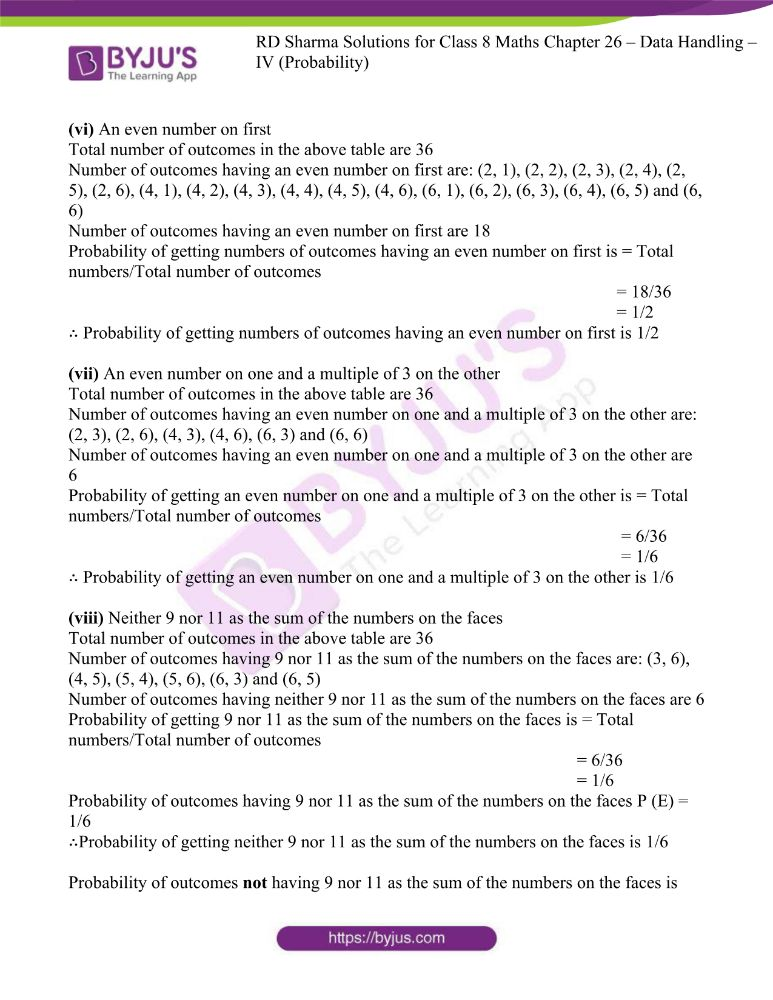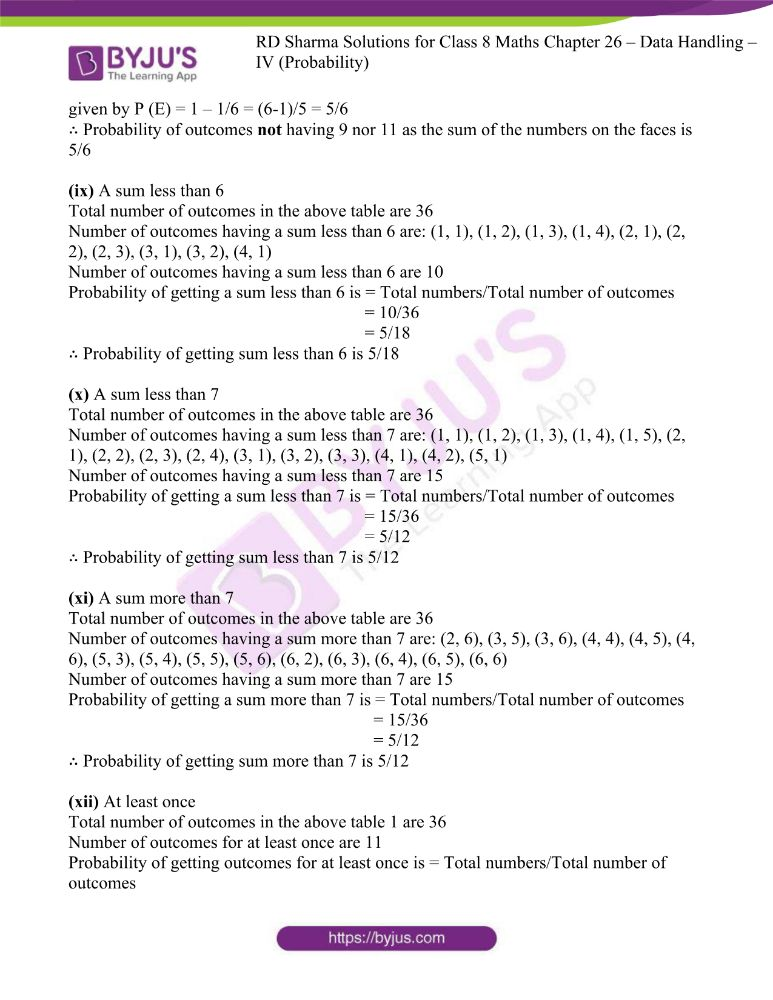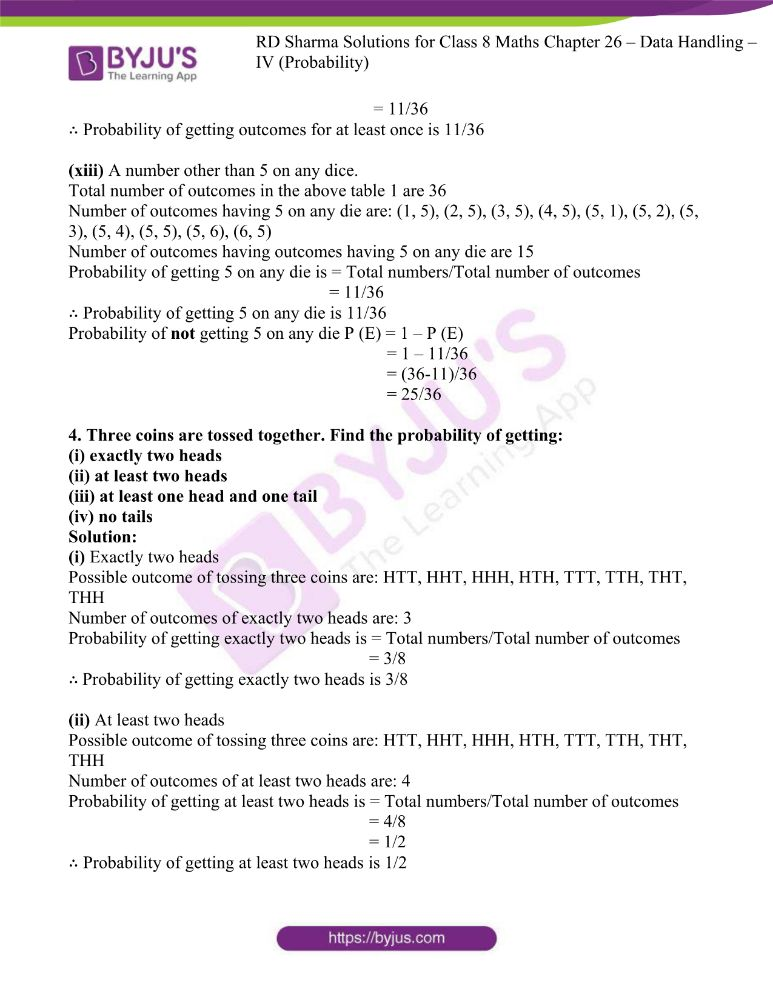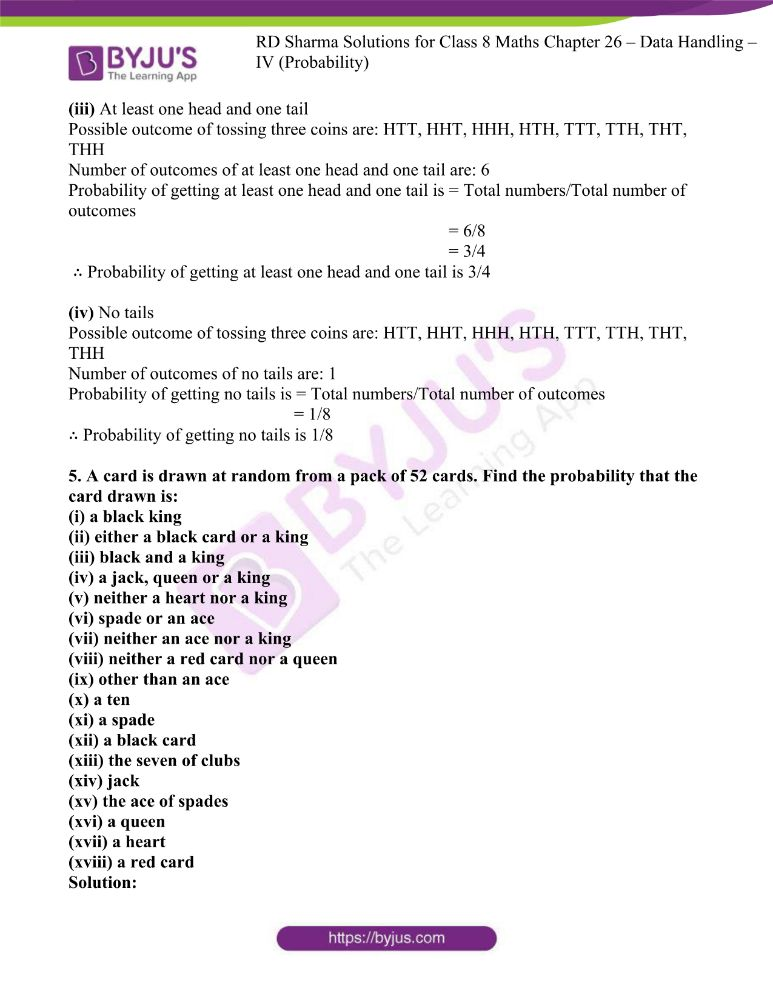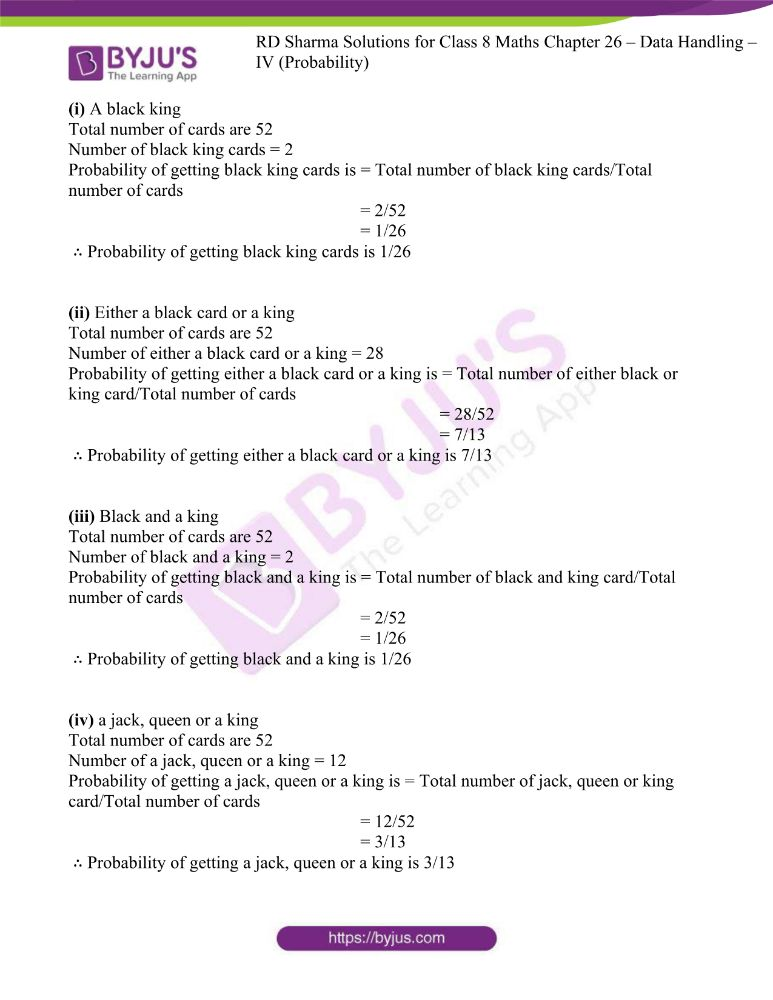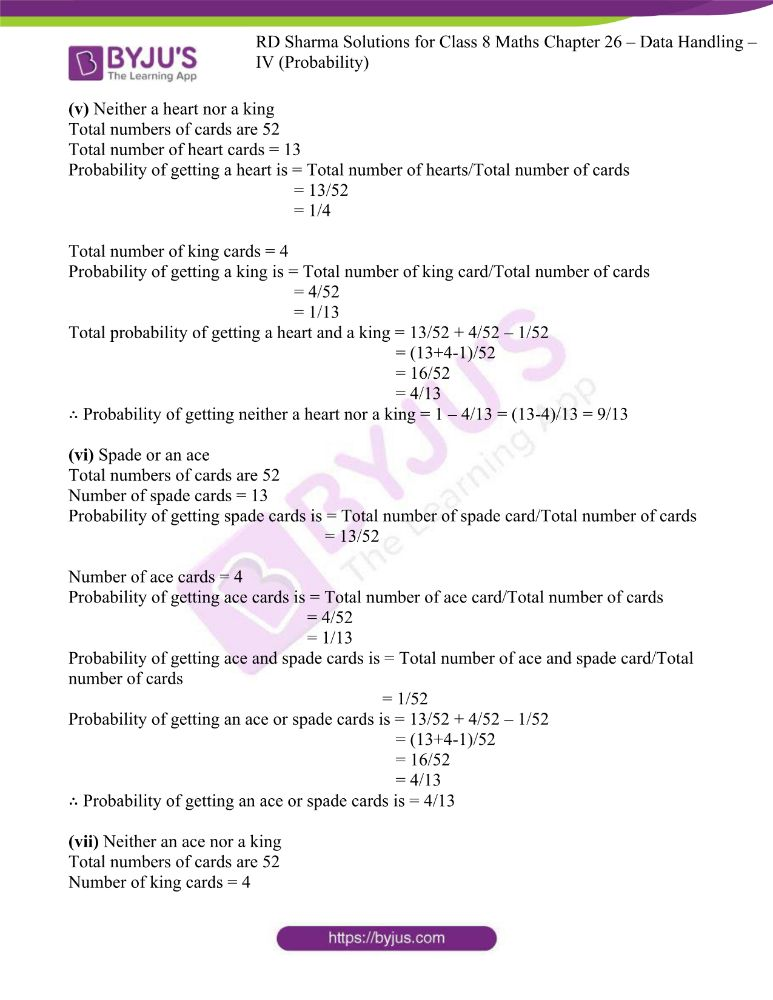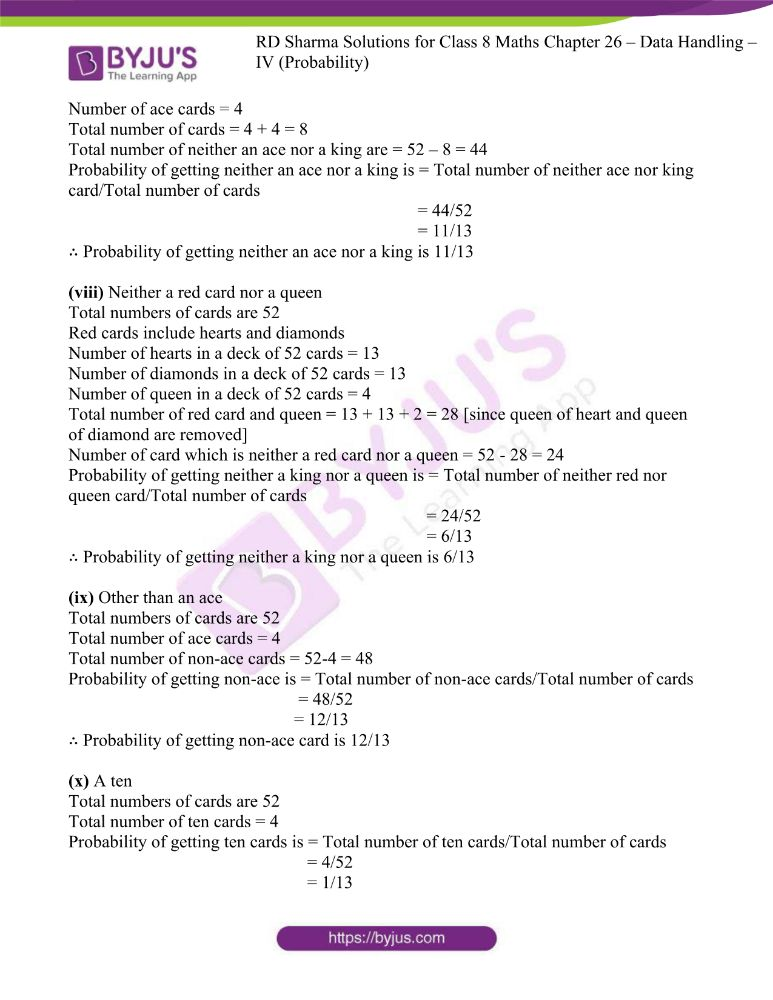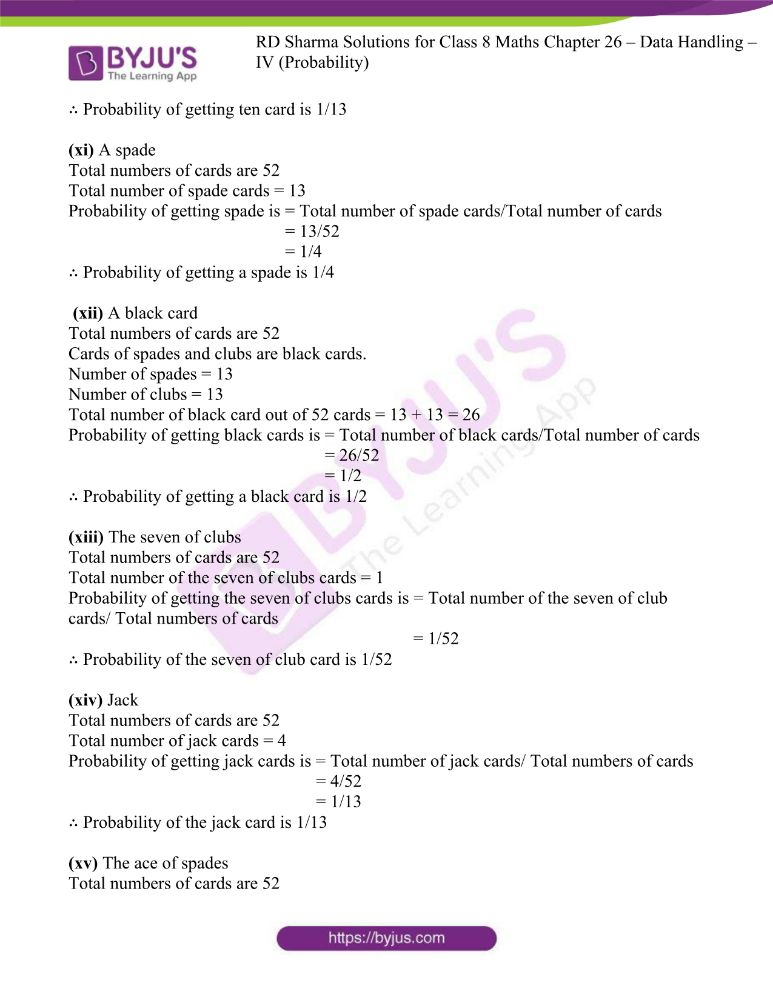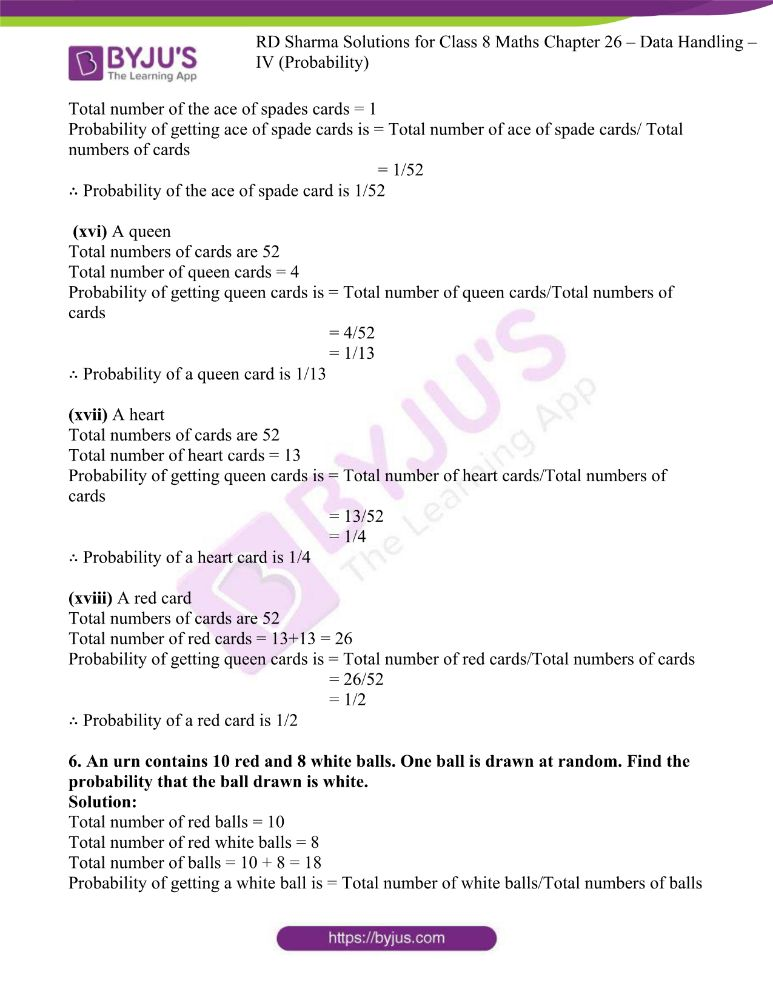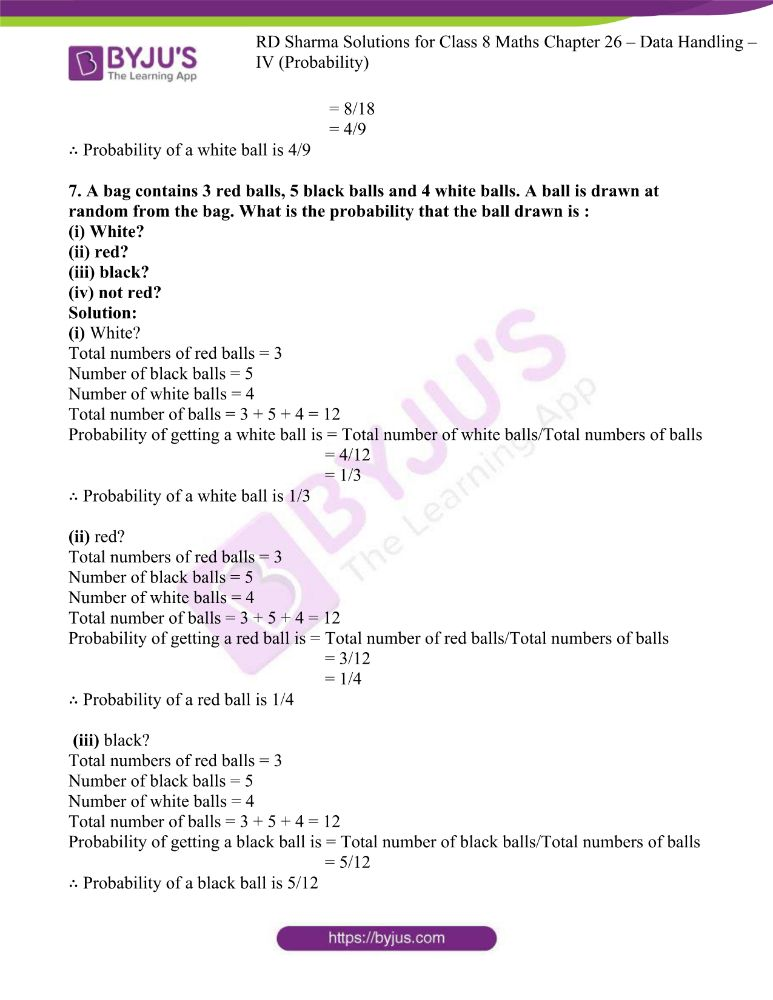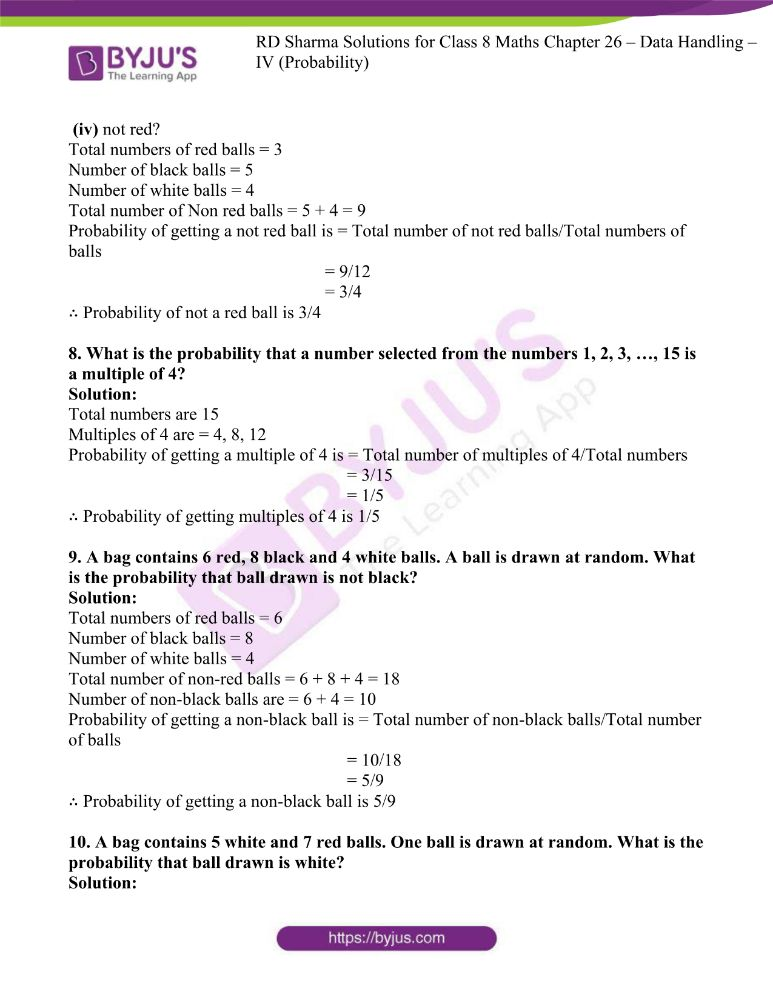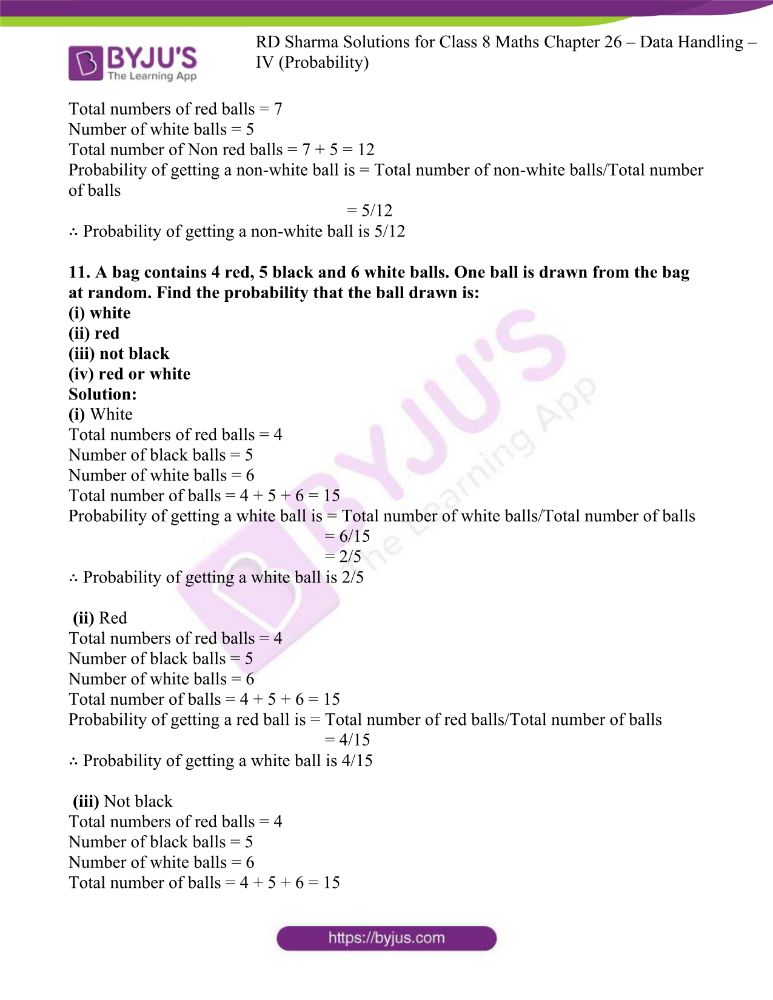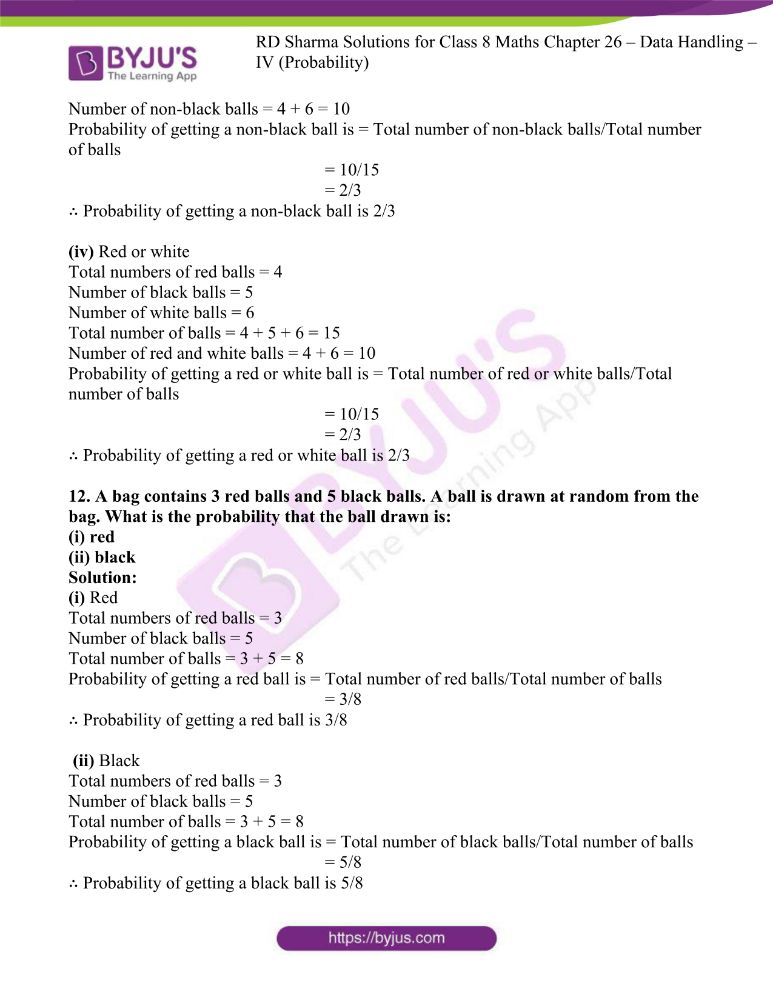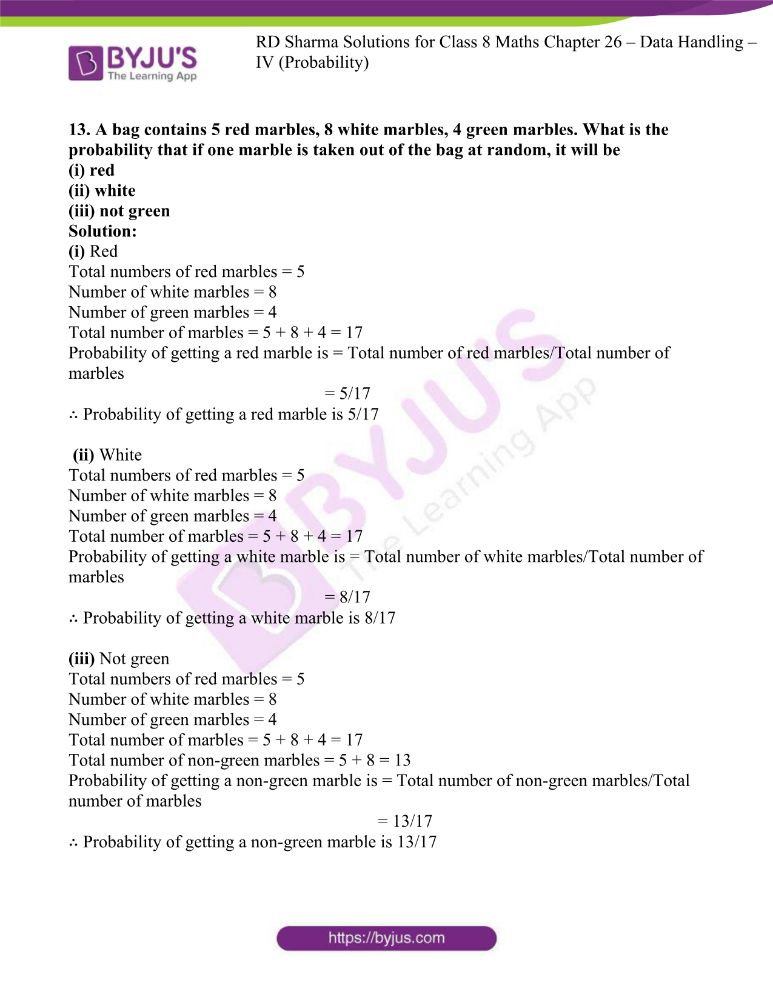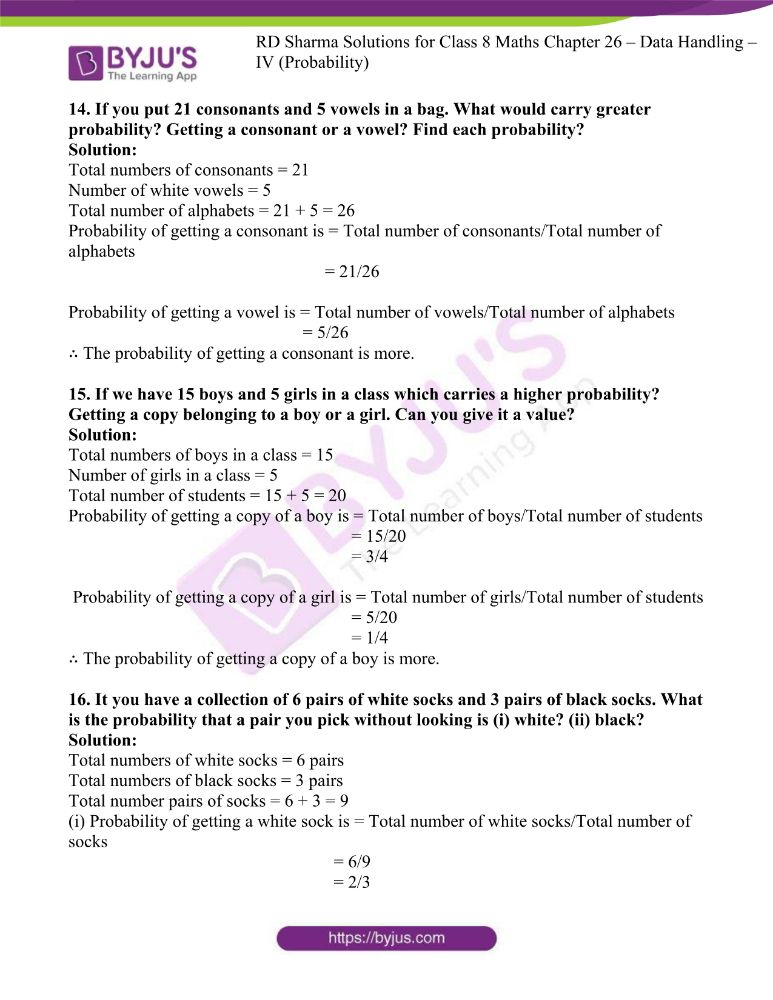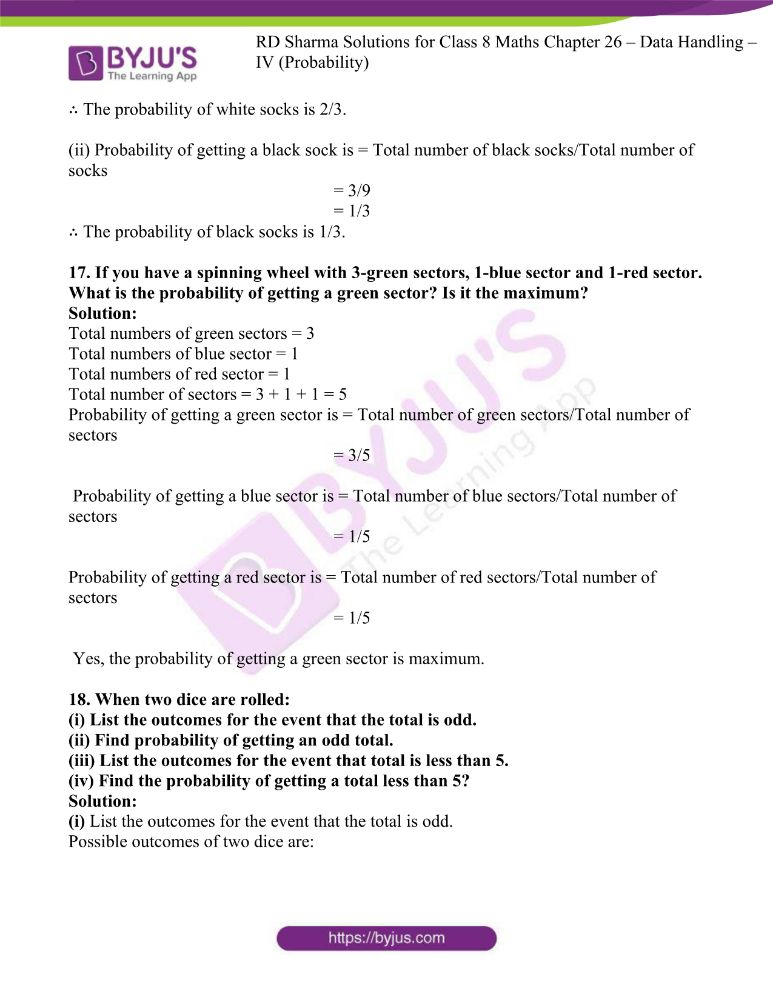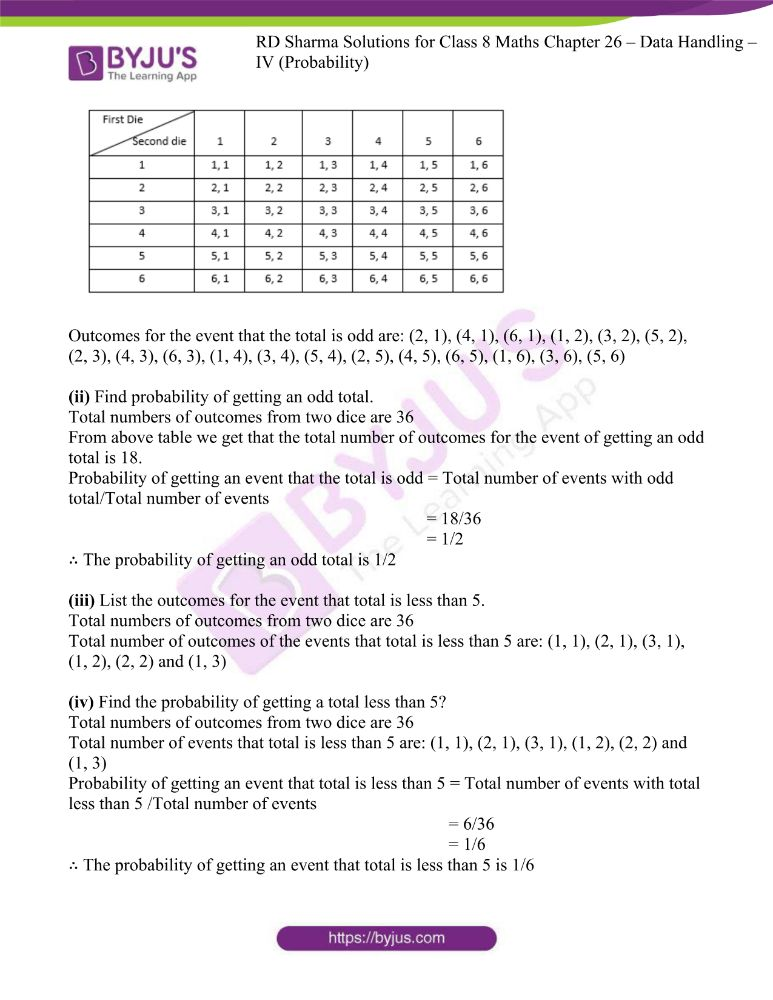### Access answers to RD Sharma Maths Solutions For Class 8 Chapter 26 Data Handling – IV (Probability)

EXERCISE 26.1 PAGE NO: 26.14

1. The probability that it will rain tomorrow is 0.85. What is the probability that it will not rain tomorrow?

Solution:

Let event of raining tomorrow be P (A)

The probability of raining tomorrow is P (A) = 0.85

Probability of not raining is given by P (A) = 1 – P (A)

∴ Probability of not raining = P (A) = 1 – 0.85

= 0.15

2. A die thrown. Find the probability of getting:
(i) a prime number
(ii) 2 or 4
(iii) a multiple of 2 or 3

Solution:

(i) Outcomes of a die are: 1, 2, 3, 4, 5, 5 and 6

Total number of outcome = 6

Prime numbers are: 1, 3 and 5

Total number of prime numbers = 3

Probability of getting a prime number = Total prime numbers/Total number of outcomes

= 3/6

= 1/2

∴ Probability of getting a prime number = 1/2

(ii) Outcomes of a die are: 1, 2, 3, 4, 5, 5 and 6

Total number of outcome = 6

Probability of getting 2 and 4 is Total numbers/Total number of outcomes

= 2/6

= 1/3

∴ Probability of getting 2 and 4 is 1/3

(iii) Outcomes of a die are: 1, 2, 3, 4, 5, 5 and 6

Multiples of 2 and 3 are = 2, 3, 4 and 6

Total number of multiples are 4

Probability of getting a multiple of 2 or 3 is Total numbers/Total number of outcomes

= 4/6

= 2/3

∴ Probability of getting a multiple of 2 or 3 is 2/3

3. In a simultaneous throw of a pair of dice, find the probability of getting:
(i) 8 as the sum
(ii) a doublet
(iii) a doublet of prime numbers
(iv) a doublet of odd numbers
(v) a sum greater than 9
(vi) An even number on first
(vii) an even number on one and a multiple of 3 on the other
(viii) neither 9 nor 11 as the sum of the numbers on the faces
(ix) a sum less than 6
(x) a sum less than 7
(xi) a sum more than 7
(xii) at least once
(xiii) a number other than 5 on any dice.

Solution:

Let us construct a table.

Here the first number denotes the outcome of first die and second number denotes the outcome of second die.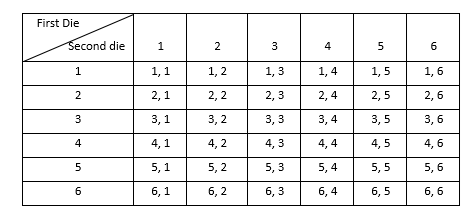(i) 8 as the sum

Total number of outcomes in the above table are 36

Number of outcomes having 8 as sum are: (6, 2), (5, 3), (4, 4), (3, 5) and (2, 6)

Therefore numbers of outcomes having 8 as sum are 5

Probability of getting numbers of outcomes having 8 as sum is = Total numbers/Total number of outcomes

= 5/36

∴ Probability of getting numbers of outcomes having 8 as sum is 5/36

(ii) a doublet

Total number of outcomes in the above table are 36

Number of outcomes as doublet are: (1, 1), (2, 2), (3, 3), (4, 4), (5, 5) and (6, 6)

Number of outcomes as doublet are 6

Probability of getting numbers of outcomes as doublet is = Total numbers/Total number of outcomes

= 6/36

= 1/6

∴ Probability of getting numbers of outcomes as doublet is 1/6

(iii) a doublet of prime numbers

Total number of outcomes in the above table are 36

Number of outcomes as doublet of prime numbers are: (1, 1), (3, 3), (5, 5)

Number of outcomes as doublet of prime numbers are 3

Probability of getting numbers of outcomes as doublet of prime numbers is = Total numbers/Total number of outcomes

= 3/36

= 1/12

∴ Probability of getting numbers of outcomes as doublet of prime numbers is 1/12

(iv) a doublet of odd numbers

Total number of outcomes in the above table are 36

Number of outcomes as doublet of odd numbers are: (1, 1), (3, 3), (5, 5)

Number of outcomes as doublet of odd numbers are 3

Probability of getting numbers of outcomes as doublet of odd numbers is = Total numbers/Total number of outcomes

= 3/36

= 1/12

∴ Probability of getting numbers of outcomes as doublet of odd numbers is 1/12

(v) a sum greater than 9

Total number of outcomes in the above table are 36

Number of outcomes having sum greater than 9 are: (4, 6), (5, 5), (5, 6), (6, 6), (6, 4), (6, 5)

Number of outcomes having sum greater than 9 are 6

Probability of getting numbers of outcomes having sum greater than 9 is = Total numbers/Total number of outcomes

= 6/36 = 1/6

∴ Probability of getting numbers of outcomes having sum greater than 9 is 1/6

(vi) An even number on first

Total number of outcomes in the above table are 36

Number of outcomes having an even number on first are: (2, 1), (2, 2), (2, 3), (2, 4), (2, 5), (2, 6), (4, 1), (4, 2), (4, 3), (4, 4), (4, 5), (4, 6), (6, 1), (6, 2), (6, 3), (6, 4), (6, 5) and (6, 6)

Number of outcomes having an even number on first are 18

Probability of getting numbers of outcomes having an even number on first is = Total numbers/Total number of outcomes

= 18/36

= 1/2

∴ Probability of getting numbers of outcomes having an even number on first is 1/2

(vii) An even number on one and a multiple of 3 on the other

Total number of outcomes in the above table are 36

Number of outcomes having an even number on one and a multiple of 3 on the other are: (2, 3), (2, 6), (4, 3), (4, 6), (6, 3) and (6, 6)

Number of outcomes having an even number on one and a multiple of 3 on the other are 6

Probability of getting an even number on one and a multiple of 3 on the other is = Total numbers/Total number of outcomes

= 6/36

= 1/6

∴ Probability of getting an even number on one and a multiple of 3 on the other is 1/6

(viii) Neither 9 nor 11 as the sum of the numbers on the faces

Total number of outcomes in the above table are 36

Number of outcomes having 9 nor 11 as the sum of the numbers on the faces are: (3, 6), (4, 5), (5, 4), (5, 6), (6, 3) and (6, 5)

Number of outcomes having neither 9 nor 11 as the sum of the numbers on the faces are 6

Probability of getting 9 nor 11 as the sum of the numbers on the faces is = Total numbers/Total number of outcomes

= 6/36

= 1/6

Probability of outcomes having 9 nor 11 as the sum of the numbers on the faces P (E) = 1/6

∴Probability of getting neither 9 nor 11 as the sum of the numbers on the faces is 1/6

Probability of outcomes not having 9 nor 11 as the sum of the numbers on the faces is given by P (E) = 1 – 1/6 = (6-1)/5 = 5/6

∴ Probability of outcomes not having 9 nor 11 as the sum of the numbers on the faces is 5/6

(ix) A sum less than 6

Total number of outcomes in the above table are 36

Number of outcomes having a sum less than 6 are: (1, 1), (1, 2), (1, 3), (1, 4), (2, 1), (2, 2), (2, 3), (3, 1), (3, 2), (4, 1)

Number of outcomes having a sum less than 6 are 10

Probability of getting a sum less than 6 is = Total numbers/Total number of outcomes

= 10/36

= 5/18

∴ Probability of getting sum less than 6 is 5/18

(x) A sum less than 7

Total number of outcomes in the above table are 36

Number of outcomes having a sum less than 7 are: (1, 1), (1, 2), (1, 3), (1, 4), (1, 5), (2, 1), (2, 2), (2, 3), (2, 4), (3, 1), (3, 2), (3, 3), (4, 1), (4, 2), (5, 1)

Number of outcomes having a sum less than 7 are 15

Probability of getting a sum less than 7 is = Total numbers/Total number of outcomes

= 15/36

= 5/12

∴ Probability of getting sum less than 7 is 5/12

(xi) A sum more than 7

Total number of outcomes in the above table are 36

Number of outcomes having a sum more than 7 are: (2, 6), (3, 5), (3, 6), (4, 4), (4, 5), (4, 6), (5, 3), (5, 4), (5, 5), (5, 6), (6, 2), (6, 3), (6, 4), (6, 5), (6, 6)

Number of outcomes having a sum more than 7 are 15

Probability of getting a sum more than 7 is = Total numbers/Total number of outcomes

= 15/36

= 5/12

∴ Probability of getting sum more than 7 is 5/12

(xii) At least once

Total number of outcomes in the above table 1 are 36

Number of outcomes for at least once are 11

Probability of getting outcomes for at least once is = Total numbers/Total number of outcomes

= 11/36

∴ Probability of getting outcomes for at least once is 11/36

(xiii) A number other than 5 on any dice.

Total number of outcomes in the above table 1 are 36

Number of outcomes having 5 on any die are: (1, 5), (2, 5), (3, 5), (4, 5), (5, 1), (5, 2), (5, 3), (5, 4), (5, 5), (5, 6), (6, 5)

Number of outcomes having outcomes having 5 on any die are 15

Probability of getting 5 on any die is = Total numbers/Total number of outcomes

= 11/36

∴ Probability of getting 5 on any die is 11/36

Probability of not getting 5 on any die P (E) = 1 – P (E)

= 1 – 11/36

= (36-11)/36

= 25/36

4. Three coins are tossed together. Find the probability of getting:
(iii) at least one head and one tail
(iv) no tails

Solution:

Possible outcome of tossing three coins are: HTT, HHT, HHH, HTH, TTT, TTH, THT, THH

Number of outcomes of exactly two heads are: 3

Probability of getting exactly two heads is = Total numbers/Total number of outcomes

= 3/8

∴ Probability of getting exactly two heads is 3/8

Possible outcome of tossing three coins are: HTT, HHT, HHH, HTH, TTT, TTH, THT, THH

Number of outcomes of at least two heads are: 4

Probability of getting at least two heads is = Total numbers/Total number of outcomes

= 4/8

= 1/2

∴ Probability of getting at least two heads is 1/2

(iii) At least one head and one tail

Possible outcome of tossing three coins are: HTT, HHT, HHH, HTH, TTT, TTH, THT, THH

Number of outcomes of at least one head and one tail are: 6

Probability of getting at least one head and one tail is = Total numbers/Total number of outcomes

= 6/8

= 3/4

∴ Probability of getting at least one head and one tail is 3/4

(iv) No tails

Possible outcome of tossing three coins are: HTT, HHT, HHH, HTH, TTT, TTH, THT, THH

Number of outcomes of no tails are: 1

Probability of getting no tails is = Total numbers/Total number of outcomes

= 1/8

∴ Probability of getting no tails is 1/8

5. A card is drawn at random from a pack of 52 cards. Find the probability that the card drawn is:
(i) a black king
(ii) either a black card or a king
(iii) black and a king
(iv) a jack, queen or a king
(v) neither a heart nor a king
(vii) neither an ace nor a king
(viii) neither a red card nor a queen
(ix) other than an ace
(x) a ten
(xii) a black card
(xiii) the seven of clubs
(xiv) jack
(xvi) a queen
(xvii) a heart
(xviii) a red card

Solution:

(i) A black king

Total number of cards are 52

Number of black king cards = 2

Probability of getting black king cards is = Total number of black king cards/Total number of cards

= 2/52

= 1/26

∴ Probability of getting black king cards is 1/26

(ii) Either a black card or a king

Total number of cards are 52

Number of either a black card or a king = 28

Probability of getting either a black card or a king is = Total number of either black or king card/Total number of cards

= 28/52

= 7/13

∴ Probability of getting either a black card or a king is 7/13

(iii) Black and a king

Total number of cards are 52

Number of black and a king = 2

Probability of getting black and a king is = Total number of black and king card/Total number of cards

= 2/52

= 1/26

∴ Probability of getting black and a king is 1/26

(iv) a jack, queen or a king

Total number of cards are 52

Number of a jack, queen or a king = 12

Probability of getting a jack, queen or a king is = Total number of jack, queen or king card/Total number of cards

= 12/52

= 3/13

∴ Probability of getting a jack, queen or a king is 3/13

(v) Neither a heart nor a king

Total numbers of cards are 52

Total number of heart cards = 13

Probability of getting a heart is = Total number of hearts/Total number of cards

= 13/52

= 1/4

Total number of king cards = 4

Probability of getting a king is = Total number of king card/Total number of cards

= 4/52

= 1/13

Total probability of getting a heart and a king = 13/52 + 4/52 – 1/52

= (13+4-1)/52

= 16/52

= 4/13

∴ Probability of getting neither a heart nor a king = 1 – 4/13 = (13-4)/13 = 9/13

Total numbers of cards are 52

Number of spade cards = 13

Probability of getting spade cards is = Total number of spade card/Total number of cards

= 13/52

Number of ace cards = 4

Probability of getting ace cards is = Total number of ace card/Total number of cards

= 4/52

= 1/13

Probability of getting ace and spade cards is = Total number of ace and spade card/Total number of cards

= 1/52

Probability of getting an ace or spade cards is = 13/52 + 4/52 – 1/52

= (13+4-1)/52

= 16/52

= 4/13

∴ Probability of getting an ace or spade cards is = 4/13

(vii) Neither an ace nor a king

Total numbers of cards are 52

Number of king cards = 4

Number of ace cards = 4

Total number of cards = 4 + 4 = 8

Total number of neither an ace nor a king are = 52 – 8 = 44

Probability of getting neither an ace nor a king is = Total number of neither ace nor king card/Total number of cards

= 44/52

= 11/13

∴ Probability of getting neither an ace nor a king is 11/13

(viii) Neither a red card nor a queen

Total numbers of cards are 52

Red cards include hearts and diamonds

Number of hearts in a deck of 52 cards = 13

Number of diamonds in a deck of 52 cards = 13

Number of queen in a deck of 52 cards = 4

Total number of red card and queen = 13 + 13 + 2 = 28 [since queen of heart and queen of diamond are removed]

Number of card which is neither a red card nor a queen = 52 – 28 = 24

Probability of getting neither a king nor a queen is = Total number of neither red nor queen card/Total number of cards

= 24/52

= 6/13

∴ Probability of getting neither a king nor a queen is 6/13

(ix) Other than an ace

Total numbers of cards are 52

Total number of ace cards = 4

Total number of non-ace cards = 52-4 = 48

Probability of getting non-ace is = Total number of non-ace cards/Total number of cards

= 48/52

= 12/13

∴ Probability of getting non-ace card is 12/13

(x) A ten

Total numbers of cards are 52

Total number of ten cards = 4

Probability of getting ten cards is = Total number of ten cards/Total number of cards

= 4/52

= 1/13

∴ Probability of getting ten card is 1/13

Total numbers of cards are 52

Total number of spade cards = 13

Probability of getting spade is = Total number of spade cards/Total number of cards

= 13/52

= 1/4

∴ Probability of getting a spade is 1/4

(xii) A black card

Total numbers of cards are 52

Cards of spades and clubs are black cards.

Number of clubs = 13

Total number of black card out of 52 cards = 13 + 13 = 26

Probability of getting black cards is = Total number of black cards/Total number of cards

= 26/52

= 1/2

∴ Probability of getting a black card is 1/2

(xiii) The seven of clubs

Total numbers of cards are 52

Total number of the seven of clubs cards = 1

Probability of getting the seven of clubs cards is = Total number of the seven of club cards/ Total numbers of cards

= 1/52

∴ Probability of the seven of club card is 1/52

(xiv) Jack

Total numbers of cards are 52

Total number of jack cards = 4

Probability of getting jack cards is = Total number of jack cards/ Total numbers of cards

= 4/52

= 1/13

∴ Probability of the jack card is 1/13

Total numbers of cards are 52

Total number of the ace of spades cards = 1

Probability of getting ace of spade cards is = Total number of ace of spade cards/ Total numbers of cards

= 1/52

∴ Probability of the ace of spade card is 1/52

(xvi) A queen

Total numbers of cards are 52

Total number of queen cards = 4

Probability of getting queen cards is = Total number of queen cards/Total numbers of cards

= 4/52

= 1/13

∴ Probability of a queen card is 1/13

(xvii) A heart

Total numbers of cards are 52

Total number of heart cards = 13

Probability of getting queen cards is = Total number of heart cards/Total numbers of cards

= 13/52

= 1/4

∴ Probability of a heart card is 1/4

(xviii) A red card

Total numbers of cards are 52

Total number of red cards = 13+13 = 26

Probability of getting queen cards is = Total number of red cards/Total numbers of cards

= 26/52

= 1/2

∴ Probability of a red card is 1/2

6. An urn contains 10 red and 8 white balls. One ball is drawn at random. Find the probability that the ball drawn is white.

Solution:

Total number of red balls = 10

Total number of red white balls = 8

Total number of balls = 10 + 8 = 18

Probability of getting a white ball is = Total number of white balls/Total numbers of balls

= 8/18

= 4/9

∴ Probability of a white ball is 4/9

7. A bag contains 3 red balls, 5 black balls and 4 white balls. A ball is drawn at random from the bag. What is the probability that the ball drawn is :
(i) White?
(ii) red?
(iii) black?
(iv) not red?

Solution:

(i) White?

Total numbers of red balls = 3

Number of black balls = 5

Number of white balls = 4

Total number of balls = 3 + 5 + 4 = 12

Probability of getting a white ball is = Total number of white balls/Total numbers of balls

= 4/12

= 1/3

∴ Probability of a white ball is 1/3

(ii) red?

Total numbers of red balls = 3

Number of black balls = 5

Number of white balls = 4

Total number of balls = 3 + 5 + 4 = 12

Probability of getting a red ball is = Total number of red balls/Total numbers of balls

= 3/12

= 1/4

∴ Probability of a red ball is 1/4

(iii) black?

Total numbers of red balls = 3

Number of black balls = 5

Number of white balls = 4

Total number of balls = 3 + 5 + 4 = 12

Probability of getting a black ball is = Total number of black balls/Total numbers of balls

= 5/12

∴ Probability of a black ball is 5/12

(iv) not red?

Total numbers of red balls = 3

Number of black balls = 5

Number of white balls = 4

Total number of Non red balls = 5 + 4 = 9

Probability of getting a not red ball is = Total number of not red balls/Total numbers of balls

= 9/12

= 3/4

∴ Probability of not a red ball is 3/4

8. What is the probability that a number selected from the numbers 1, 2, 3, …, 15 is a multiple of 4?

Solution:

Total numbers are 15

Multiples of 4 are = 4, 8, 12

Probability of getting a multiple of 4 is = Total number of multiples of 4/Total numbers

= 3/15

= 1/5

∴ Probability of getting multiples of 4 is 1/5

9. A bag contains 6 red, 8 black and 4 white balls. A ball is drawn at random. What is the probability that ball drawn is not black?

Solution:

Total numbers of red balls = 6

Number of black balls = 8

Number of white balls = 4

Total number of non-red balls = 6 + 8 + 4 = 18

Number of non-black balls are = 6 + 4 = 10

Probability of getting a non-black ball is = Total number of non-black balls/Total number of balls

= 10/18

= 5/9

∴ Probability of getting a non-black ball is 5/9

10. A bag contains 5 white and 7 red balls. One ball is drawn at random. What is the probability that ball drawn is white?

Solution:

Total numbers of red balls = 7

Number of white balls = 5

Total number of Non red balls = 7 + 5 = 12

Probability of getting a non-white ball is = Total number of non-white balls/Total number of balls

= 5/12

∴ Probability of getting a non-white ball is 5/12

11. A bag contains 4 red, 5 black and 6 white balls. One ball is drawn from the bag at random. Find the probability that the ball drawn is:
(i) white
(ii) red
(iii) not black
(iv) red or white

Solution:

(i) White

Total numbers of red balls = 4

Number of black balls = 5

Number of white balls = 6

Total number of balls = 4 + 5 + 6 = 15

Probability of getting a white ball is = Total number of white balls/Total number of balls

= 6/15

= 2/5

∴ Probability of getting a white ball is 2/5

(ii) Red

Total numbers of red balls = 4

Number of black balls = 5

Number of white balls = 6

Total number of balls = 4 + 5 + 6 = 15

Probability of getting a red ball is = Total number of red balls/Total number of balls

= 4/15

∴ Probability of getting a white ball is 4/15

(iii) Not black

Total numbers of red balls = 4

Number of black balls = 5

Number of white balls = 6

Total number of balls = 4 + 5 + 6 = 15

Number of non-black balls = 4 + 6 = 10

Probability of getting a non-black ball is = Total number of non-black balls/Total number of balls

= 10/15

= 2/3

∴ Probability of getting a non-black ball is 2/3

(iv) Red or white

Total numbers of red balls = 4

Number of black balls = 5

Number of white balls = 6

Total number of balls = 4 + 5 + 6 = 15

Number of red and white balls = 4 + 6 = 10

Probability of getting a red or white ball is = Total number of red or white balls/Total number of balls

= 10/15

= 2/3

∴ Probability of getting a red or white ball is 2/3

12. A bag contains 3 red balls and 5 black balls. A ball is drawn at random from the bag. What is the probability that the ball drawn is:
(i) red
(ii) black

Solution:

(i) Red

Total numbers of red balls = 3

Number of black balls = 5

Total number of balls = 3 + 5 = 8

Probability of getting a red ball is = Total number of red balls/Total number of balls

= 3/8

∴ Probability of getting a red ball is 3/8

(ii) Black

Total numbers of red balls = 3

Number of black balls = 5

Total number of balls = 3 + 5 = 8

Probability of getting a black ball is = Total number of black balls/Total number of balls

= 5/8

∴ Probability of getting a black ball is 5/8

13. A bag contains 5 red marbles, 8 white marbles, 4 green marbles. What is the probability that if one marble is taken out of the bag at random, it will be
(i) red
(ii) white
(iii) not green

Solution:

(i) Red

Total numbers of red marbles = 5

Number of white marbles = 8

Number of green marbles = 4

Total number of marbles = 5 + 8 + 4 = 17

Probability of getting a red marble is = Total number of red marbles/Total number of marbles

= 5/17

∴ Probability of getting a red marble is 5/17

(ii) White

Total numbers of red marbles = 5

Number of white marbles = 8

Number of green marbles = 4

Total number of marbles = 5 + 8 + 4 = 17

Probability of getting a white marble is = Total number of white marbles/Total number of marbles

= 8/17

∴ Probability of getting a white marble is 8/17

(iii) Not green

Total numbers of red marbles = 5

Number of white marbles = 8

Number of green marbles = 4

Total number of marbles = 5 + 8 + 4 = 17

Total number of non-green marbles = 5 + 8 = 13

Probability of getting a non-green marble is = Total number of non-green marbles/Total number of marbles

= 13/17

∴ Probability of getting a non-green marble is 13/17

14. If you put 21 consonants and 5 vowels in a bag. What would carry greater probability? Getting a consonant or a vowel? Find each probability?

Solution:

Total numbers of consonants = 21

Number of white vowels = 5

Total number of alphabets = 21 + 5 = 26

Probability of getting a consonant is = Total number of consonants/Total number of alphabets

= 21/26

Probability of getting a vowel is = Total number of vowels/Total number of alphabets

= 5/26

∴ The probability of getting a consonant is more.

15. If we have 15 boys and 5 girls in a class which carries a higher probability? Getting a copy belonging to a boy or a girl. Can you give it a value?

Solution:

Total numbers of boys in a class = 15

Number of girls in a class = 5

Total number of students = 15 + 5 = 20

Probability of getting a copy of a boy is = Total number of boys/Total number of students

= 15/20

= 3/4

Probability of getting a copy of a girl is = Total number of girls/Total number of students

= 5/20

= 1/4

∴ The probability of getting a copy of a boy is more.

16. It you have a collection of 6 pairs of white socks and 3 pairs of black socks. What is the probability that a pair you pick without looking is (i) white? (ii) black?

Solution:

Total numbers of white socks = 6 pairs

Total numbers of black socks = 3 pairs

Total number pairs of socks = 6 + 3 = 9

(i) Probability of getting a white sock is = Total number of white socks/Total number of socks

= 6/9

= 2/3

∴ The probability of white socks is 2/3.

(ii) Probability of getting a black sock is = Total number of black socks/Total number of socks

= 3/9

= 1/3

∴ The probability of black socks is 1/3.

17. If you have a spinning wheel with 3-green sectors, 1-blue sector and 1-red sector. What is the probability of getting a green sector? Is it the maximum?

Solution:

Total numbers of green sectors = 3

Total numbers of blue sector = 1

Total numbers of red sector = 1

Total number of sectors = 3 + 1 + 1 = 5

Probability of getting a green sector is = Total number of green sectors/Total number of sectors

= 3/5

Probability of getting a blue sector is = Total number of blue sectors/Total number of sectors

= 1/5

Probability of getting a red sector is = Total number of red sectors/Total number of sectors

= 1/5

Yes, the probability of getting a green sector is maximum.

18. When two dice are rolled:
(i) List the outcomes for the event that the total is odd.
(ii) Find probability of getting an odd total.
(iii) List the outcomes for the event that total is less than 5.
(iv) Find the probability of getting a total less than 5?

Solution:

(i) List the outcomes for the event that the total is odd.

Possible outcomes of two dice are:Outcomes for the event that the total is odd are: (2, 1), (4, 1), (6, 1), (1, 2), (3, 2), (5, 2), (2, 3), (4, 3), (6, 3), (1, 4), (3, 4), (5, 4), (2, 5), (4, 5), (6, 5), (1, 6), (3, 6), (5, 6)

(ii) Find probability of getting an odd total.

Total numbers of outcomes from two dice are 36

From above table we get that the total number of outcomes for the event of getting an odd total is 18.

Probability of getting an event that the total is odd = Total number of events with odd total/Total number of events

= 18/36

= 1/2

∴ The probability of getting an odd total is 1/2

(iii) List the outcomes for the event that total is less than 5.

Total numbers of outcomes from two dice are 36

Total number of outcomes of the events that total is less than 5 are: (1, 1), (2, 1), (3, 1), (1, 2), (2, 2) and (1, 3)

(iv) Find the probability of getting a total less than 5?

Total numbers of outcomes from two dice are 36

Total number of events that total is less than 5 are: (1, 1), (2, 1), (3, 1), (1, 2), (2, 2) and (1, 3)

Probability of getting an event that total is less than 5 = Total number of events with total less than 5 /Total number of events

= 6/36

= 1/6

∴ The probability of getting an event that total is less than 5 is 1/6